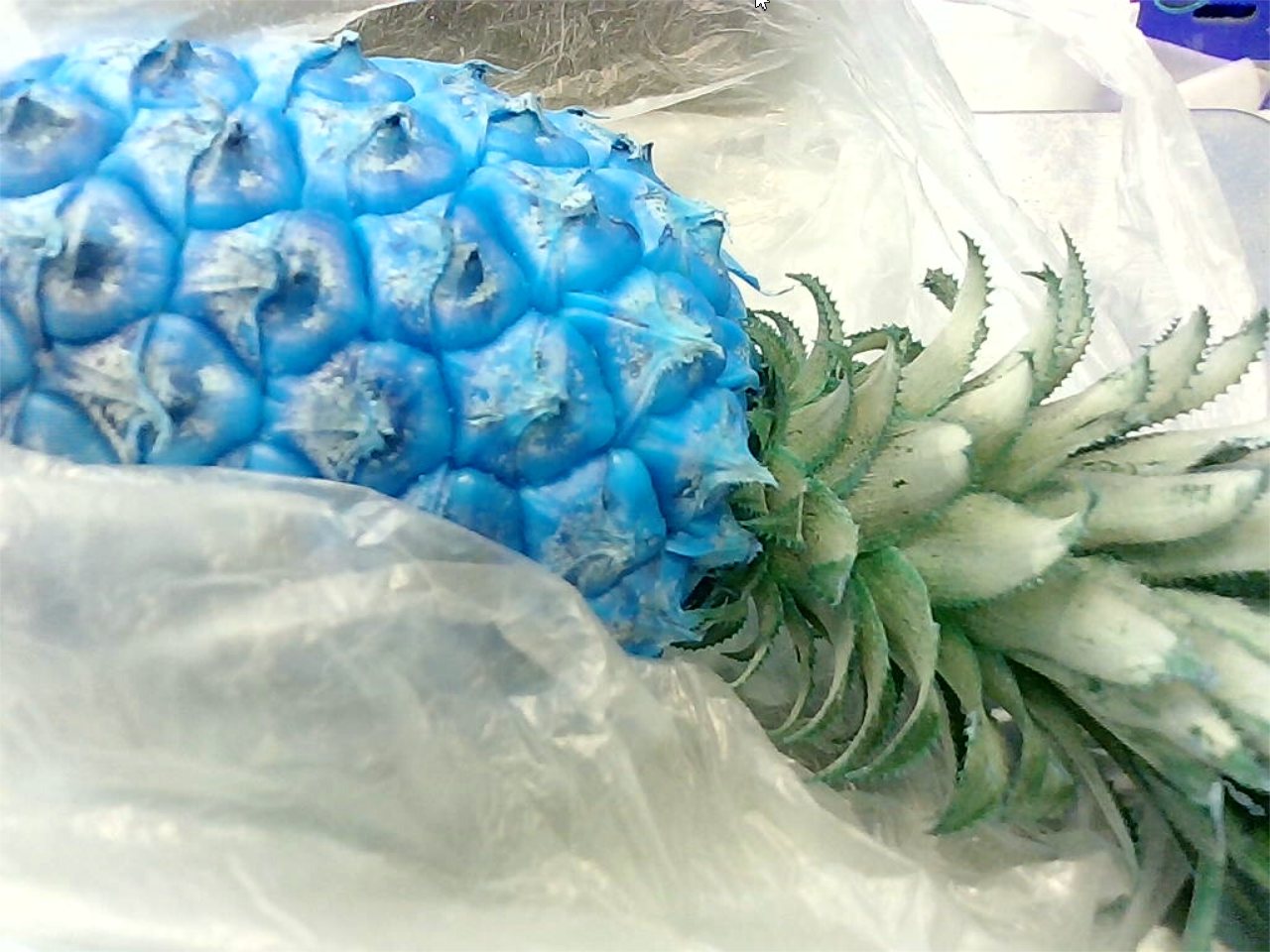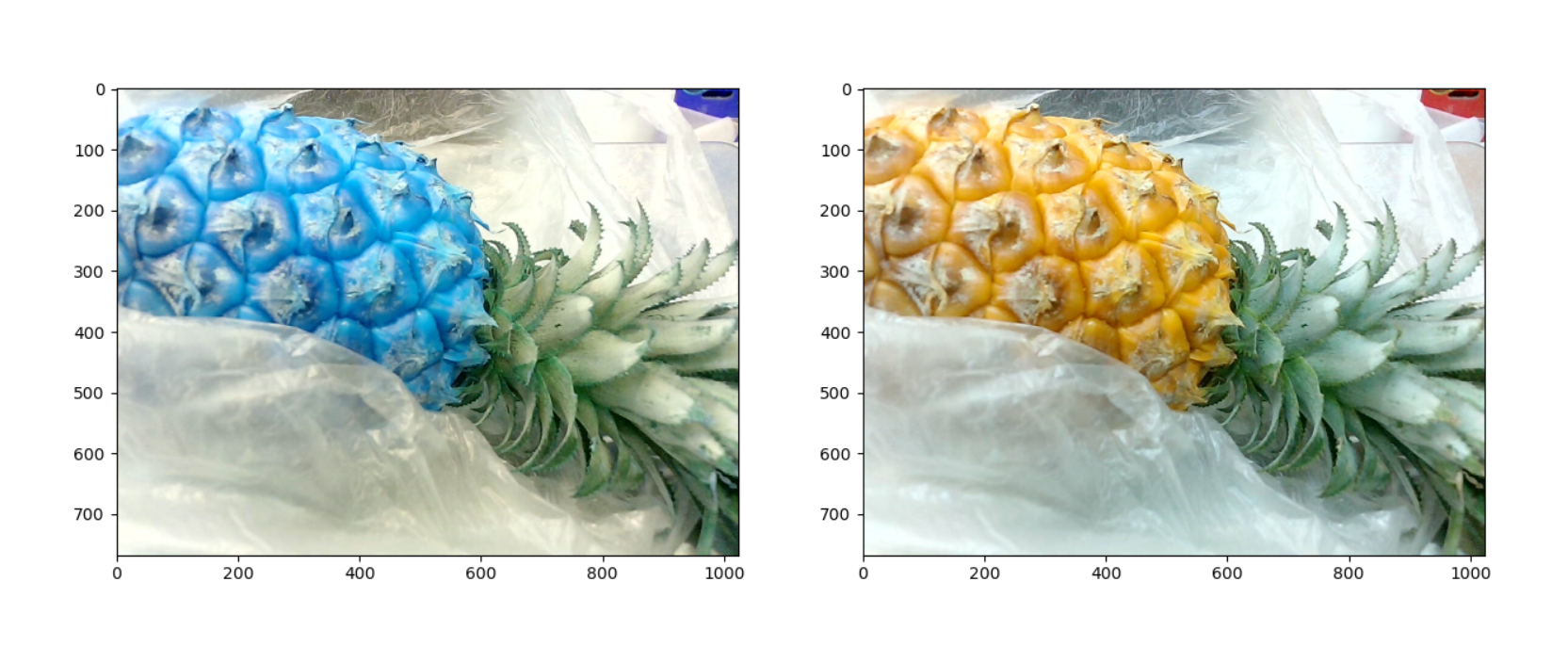# opencv讀取圖片通道以及顯示

```cpp img=cv2.imread('515.jpg') cv2.imshow("aa",img) cv2.waitKey(-1)``` 顯示結果：顯示結果是正常的。如果轉為RGB後？

```cpp import matplotlib.pyplot as plt import cv2

img=cv2.imread('515.jpg') img_rgb = cv2.cvtColor(img, cv2.COLOR_BGR2RGB) cv2.imshow("aa",img_rgb) cv2.waitKey(-1) ```然後卻變成BGR了！為什麼呢？難道cv2讀取的通達不是BGR嗎？ 我再換一種方式，使用matplotlib.pyplot顯示圖片。 代碼：

```python img=cv2.imread('515.jpg') img_rgb = cv2.cvtColor(img, cv2.COLOR_BGR2RGB) fig, axes = plt.subplots(1, 2, figsize=(15, 15)) axes.imshow(img) axes.imshow(img_rgb) plt.show()``` 輸出結果：結論：

cv2.imread( ) 所讀取的圖像格式：每個像素為[B,G,R]的形式 cv2.imshow( ) 顯示圖像：按圖像中每個像素為[B,G,R]的規則將圖像顯示出來 所以使用cv2.imshow( )顯示圖像，我看到的是正常的圖片。 如果換成matplotlib顯示圖像？ mpimg.imread( ) 所讀取的圖像格式：每個像素為[R,G,B]的形式 plt.imshow( ) 顯示圖像：按圖像中每個像素為[R,G,B]的規則將圖像顯示出來 這時候圖像就是偏藍色的。 這也就是OpenCV讀取的圖片使用的時候，為啥要做BGR2RGB的轉換。